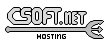Agar 1.7 Manual

SG_Node(3)

# SYNOPSIS

```#include <agar/core.h>
#include <agar/sg.h>
```

# DESCRIPTION

SG_Node is the base class for any element of a SG(3) scene. This includes geometrical elements (e.g., SG_Object(3)) as well as non-geometrical elements (e.g., SG_Light(3), SG_Camera(3)).

Nodes are organized in a tree structure, and the position of a SG_Node in relation to its parent is determined by a 4x4 transformation matrix (see M_Matrix(3)).

# INHERITANCE HIERARCHY

AG_Object(3)-> SG_Node.

# INTERFACE

void SG_RegisterClass (SG_NodeOps *ops)

void SG_NodeInit (void *node, const char *name, const SG_NodeOps *ops, Uint flags)

void * SG_NodeAdd (void *pnode, const char *name, const SG_NodeOps *ops, Uint flags)

void SG_NodeAttach (void *pnode, void *node)

void SG_NodeDetach (void *pnode, void *node)

void SG_NodeDraw (SG *sg, SG_Node *node, SG_View *view)

The SG_RegisterClass() function registers a new node class, described by the given SG_NodeOps structure.

The SG_NodeInit() function initializes the given SG_Node structure. It is usually invoked from node constructor functions. The name argument is a string identifier for the node. ops points to the SG_NodeOps structure which contains class information. The flags argument should be 0. The SG_NodeAdd() variant also allocates, initializes and attaches the node to a parent node.

The SG_NodeAttach() and SG_NodeDetach() functions attach/detach a node to/from a given parent.

The SG_NodeDraw() function is used to render a node to the display. It is normally only invoked from the draw operation of SG_View(3) (or another visualization widget derived from SG_View). SG_NodeDraw() assumes that the node's transformation matrix has already been applied to the current viewing matrix.

# NODE TRANSFORMATIONS

The following calls multiply a node's transformation matrix T with a translation, scaling or rotation matrix. Note that the T matrix may also be manipulated directly with the M_Matrix(3) interface.

void SG_Identity (SG_Node *node)

void SG_Translate (SG_Node *node, M_Real x, M_Real y, M_Real z)

void SG_Translatev (SG_Node *node, M_Vector3 v)

void SG_TranslateX (SG_Node *node, M_Real x)

void SG_TranslateY (SG_Node *node, M_Real y)

void SG_TranslateZ (SG_Node *node, M_Real z)

void SG_Scale (SG_Node *node, M_Real s)

void SG_Rotatev (SG_Node *node, M_Real theta, M_Vector3 axis)

void SG_RotateI (SG_Node *node, M_Real theta)

void SG_RotateJ (SG_Node *node, M_Real theta)

void SG_RotateK (SG_Node *node, M_Real theta)

void SG_Rotatevd (SG_Node *node, M_Real degrees, M_Vector3 axis)

void SG_RotateId (SG_Node *node, M_Real degrees)

void SG_RotateJd (SG_Node *node, M_Real degrees)

void SG_RotateKd (SG_Node *node, M_Real degrees)

void SG_GetNodeTransform (void *node, M_Matrix44 *T)

void SG_GetNodeTransformInverse (void *node, M_Matrix44 *T)

M_Vector3 SG_NodePos (SG_Node *node)

M_Vector3 SG_NodeDir (SG_Node *node)

M_Real SG_NodeSize (SG_Node *node)

SG_Identity() sets the transformation matrix of the node to the identity matrix.
The SG_Translate*() functions multiply T by a translation matrix.
The SG_Scale() function multiplies T by a uniform scaling matrix.
SG_Rotate*() multiply T by a rotation matrix. Angles are given in radians, except for SG_Rotate*d() variants which accept angular arguments in degrees.
SG_Rotatev() generates a rotation of theta radians around axis.
Note that most of the preceding functions are trivial wrappers around M_Matrix(3) functions (applied to the transformation matrix T of the node).
The SG_GetNodeTransform() function returns a transformation matrix mapping the node back to world coordinates (i.e., by computing the product of the transformation matrices of the node and its parents). SG_GetNodeTransformInverse() returns the inverse of this matrix.
The SG_NodePos() function returns a vector representing the absolute world coordinates of a node. SG_NodeDir() returns a normalized vector representing the direction of a node with respect to the world Z axis (i.e., the Z axis of the origin node). SG_NodeSize() returns the absolute scaling factor of an object.

# STRUCTURE DATA

For the SG_Node object:
 Uint flags Option flags (see FLAGS section). SG *sg Back pointer to parent SG(3) object. M_Matrix44 T Transformation matrix (relative to parent node).

# FLAGS

For the SG_Node object:
 SG_NODE_SELECTED Node is selected (e.g., for edition).

# EXAMPLES

See sg/sg_dummy.c in the Agar source distribution for an example node class implementation.www.libAgar.org is © 2022 Julien Nadeau Carriere <vedge@csoft.net>.